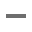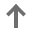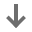# Change Signature

The Change Signature refactoring combines several different modifications that can be applied to a function signature. You can use this refactoring to:

• change the function name and return type

• add, remove, and reorder parameters

When changing a function signature, CLion searches for all usages of the function and updates all the calls, implementations, and override replacements of the function that can be safely modified to reflect the change.

## Examples

The following tables show possible ways of performing the Change Signature refactoring for code in different languages.

Before

After

//Initial function signature int exFunction2(int a, int b) { int x = a+b; return x; }; int main() { int a = 0, b = 1; int x = exFunction2(a, b); return 0; }
//Name changed, the third parameter introduced int exFunction3(int a, int b, int c) { int x = a+b; return x; }; int main() { int a = 0, b = 1; int x = exFunction3(a, b, 0); //default value '0' added return 0; }

Before

After

//This function will be turned into a block float multiply (int x, int y) { return x * y; } int main (int argc, const char * argv[]) { @autoreleasepool { float result; result = multiply( 10, 20 ); } return 0; }
int main (int argc, const char * argv[]) { @autoreleasepool { float result; result = //a block was generated ^float(int x, int y) { return x * y; }(10, 20); } return 0; }

Before

After

# This function will be renamed def fibonacci( n ): a, b = 0, 1 while b < n: print( b ) a, b = b, a+b n = int(input("n = ")) fibonacci( n )
# Function with the new name def fibonacci_numbers( n ): a, b = 0, 1 while b < n: print( b ) a, b = b, a+b n = int(input("n = ")) fibonacci_numbers( n )

Before

After

//This function wil be renamed //and a default parameter will be added function result() { } function show_result() { alert('Result: ' + result()); }
//Function with a new name and default parameter function generate_result(input = 100) { } function show_result() { alert('Result: ' + generate_result()); }

## Changing a function signature

1. Position the caret at the name of the function that you want to refactor.

2. Press Ctrl+F6. Alternatively, select Refactor | Change Signature from the main menu or from the context menu.

3. In the Change Signature dialog, make necessary changes to the function signature depending on your needs:

• Change the function name. To change the name, edit the text in the Name field.

• Manage the function parameters. To configure the parameters, use the table and the buttons in the Parameters area:

• To add a new parameter, clickand specify the new parameter's properties in the corresponding table row.

• To remove a parameter, select any row and click.

• To reorder the parameters, clickand. For example, if you want to put a certain parameter first in the list, click any of the cells in the row corresponding to that parameter, and then clickthe required number of times.

To see the expected changes and make adjustments prior to the refactoring, click Preview.

4. Click Refactor.

5. To see the expected changes and make adjustments prior to the refactoring, click Preview.